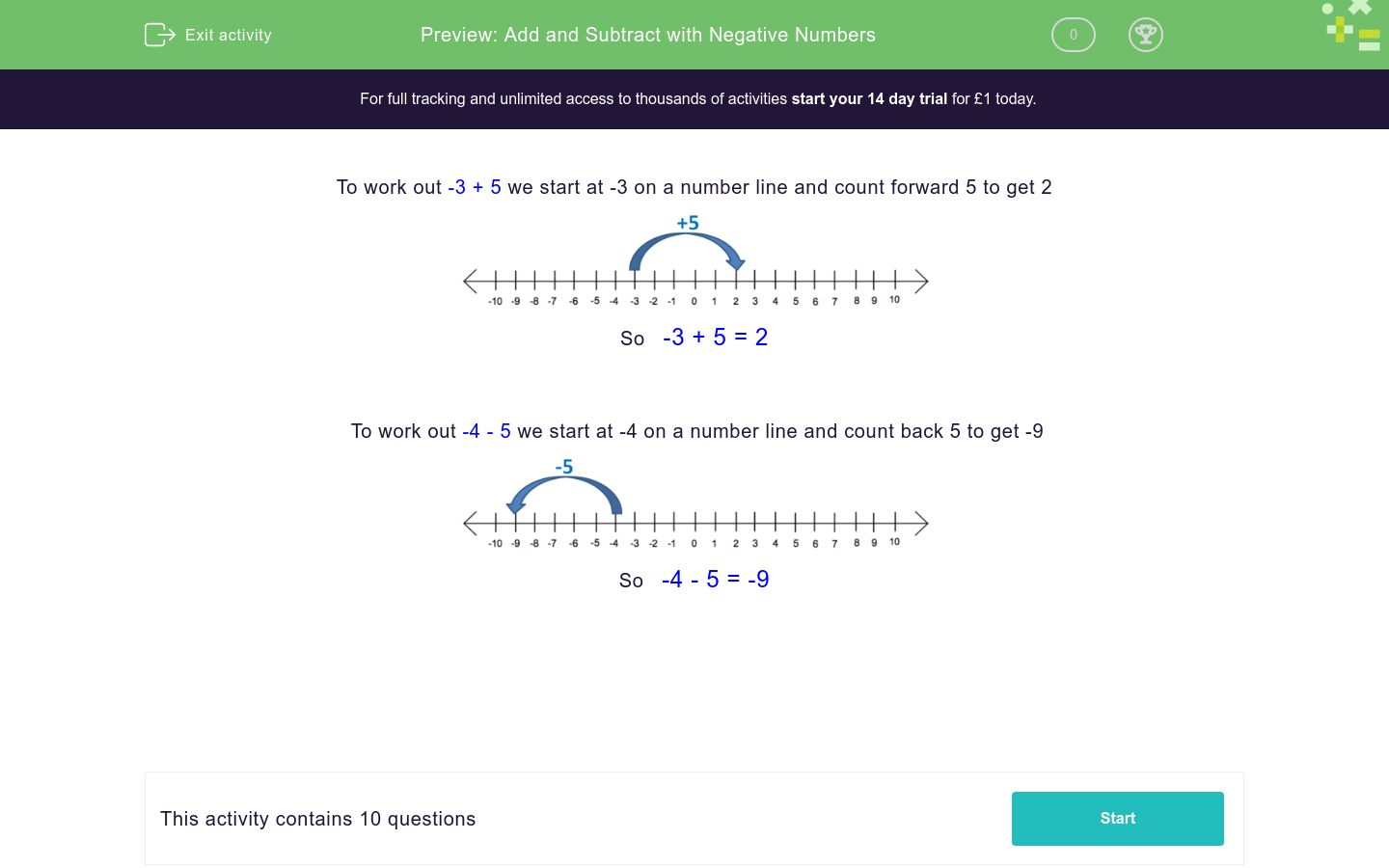# Add and Subtract with Negative Numbers

In this worksheet, students add and subtract using negative numbers by moving along a number line.Key stage:  KS 2

Curriculum topic:   Number: Number and Place Value

Curriculum subtopic:   Interpret Negative Numbers

Difficulty level:### QUESTION 1 of 10

To work out -3 + 5 we start at -3 on a number line and count forward 5 to get 2So   -3 + 5 = 2

To work out -4 - 5 we start at -4 on a number line and count back 5 to get -9So   -4 - 5 = -9

---- OR ----